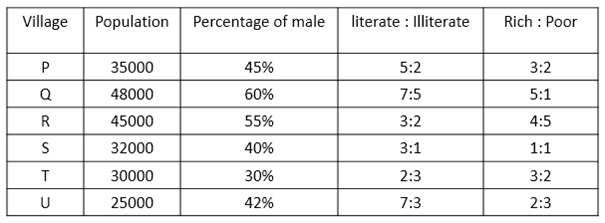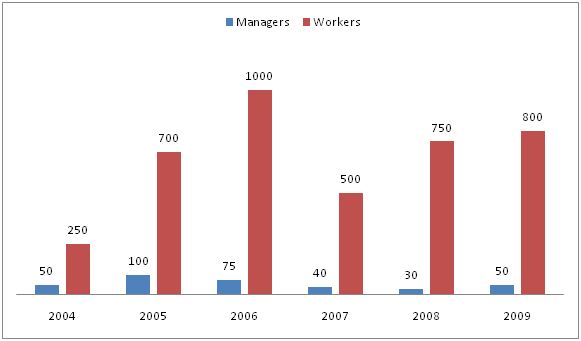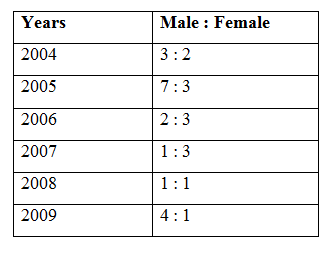# Quantitative Aptitude Questions (Data Interpretation) for SBI Clerk Mains 2018 Day-170

Dear Readers, SBI is conducting Online Examination for the recruitment of clerical cadre To enrich your preparation here we have providing new series of Data Interpretation – Quantitative Aptitude Questions. Candidates those who are appearing in SBI Clerk Mains Exams can practice these Quantitative Aptitude average questions daily and make your preparation effective.

[WpProQuiz 3161]

Click “Start Quiz” to attend these Questions and view Solutions

Directions (Q. 1 – 5): Study the following information carefully and answer the questions given below:1) If out of females of village Q, 30% are literate, then find the number of literate males of village Q?

a) 32400

b) 21340

c) 22240

d) 20040

e) None of these

2) Total number of rich population of village P is what percent of total number of females of village T?

a) 200 %

b) 100 %

c) 125 %

d) 150 %

e) 175 %

3) Find out the total number of rich population from all the given villages together?

a) 14000

b) 135000

c) 120000

d) 125000

e) None of these

4) If the ratio of literate males to literate females of village S is 2 : 3, then find the number of illiterate males of village S?

a) 3200

b) 3500

c) 3800

d) 4200

e) None of these

5) Literate population of village R is what percentage more than the poor population of village U?

a) 100 %

b) 70 %

c) 90 %

d) 80 %

e) None of these

Directions (Q. 6 – 10): Study the following information carefully and answer the questions given below.

The following bar graph represents the number of employees (managers and workers) working in a company and the table shows the ratio of male and female in 6 different years.6) Find the total number of female employees of the company over all the years together?

a) 1658

b) 1852

c) 1970

d) 1540

e) None of these

7) Total number of managers in a company is approximately what percentage of total number of workers in a company in all the given years?

a) 15 %

b) 22 %

c) 9 %

d) 4 %

e) 26 %

8) If in the year 2004, out of total male employees, 3/4th are workers, then find the ratio of male workers in 2004 to the total number of female employees in 2009?

a) 13 : 15

b) 14 : 25

c) 34 : 27

d) 27 : 34

e) None of these

9) Number of workers in 2008 is approximately what percent of number of workers in 2005?

a) 123 %

b) 95 %

c) 107 %

d) 74 %

e) 116 %

10) Find the difference between the total number of female employees in 2006 and total number of female employees in 2008.

a) 145

b) 255

c) 146

d) 256

e) None of these

Explanation:

Total number of females of village Q = (40/100)*48000 = 19200

Number of literate females = (30/100)*19200 = 5760

Total number of literate population of village Q = (7/12)*48000 = 28000

Number of literate males = 28000 – 5760 = 22240

Explanation:

Total number of rich population of village P = (3/5)*35000 = 21000

Total number of females of village T = [(100 – 30)/100]*30000 = 21000

Required percentage = (21000/21000)*100 = 100 %

Explanation:

Rich population of,

P = (3/5)*35000 = 21000

Q = (5/6)*48000 = 40000

R = (4/9)*45000 = 20000

S = (1/2)*32000 = 16000

T = (3/5)*30000 = 18000

U = (2/5)*25000 = 10000

Required sum = 21000 + 40000 + 20000 + 16000 + 18000 + 10000 = 125000

Explanation:

Number of males of village S = (40/100)*32000 = 12800

Number of literate population of village S = (¾)*32000 = 24000

Number of literate males = (2/5)*24000 = 9600

Number of illiterate males = 12800 – 9600 = 3200

Explanation:

Literate population of village R = (3/5)*45000 = 27000

Poor population of village U = (3/5)*25000 = 15000

Required percentage = [(27000 – 15000)/15000]*100 = 80 %

Explanation:

Total number of employees in 2004 = 50 + 250 = 300

Females in 2004 = (2/5)*300 = 120

Total number of employees in 2005 = 100 + 700 = 800

Females in 2005 = (3/10)*800 = 240

Total number of employees in 2006 = 75 + 1000 = 1075

Females in 2006 = (3/5)*1075 = 645

Total number of employees in 2007 = 40 + 500 = 540

Females in 2007 = (3/4)*540 = 405

Total number of employees in 2008 = 30 + 750 = 780

Females in 2008 = (1/2)*780 = 390

Total number of employees in 2009 = 50 + 800 = 850

Females in 2009 = (1/5)*850 = 170

Total number of female employees

= > 120 + 240 + 645 + 405 + 390 + 170 = 1970

Explanation:

Total number of managers = 50 + 100 + 75 + 40 + 30 + 50 = 345

Total number of workers = 250 + 700 + 1000 + 500 + 750 + 800 = 4000

Required % = (345/4000)*100 = 9 %

Explanation:

Number of male workers in 2004 = (50 + 250)*(3/5)*(3/4) = 135

Number of female employees in 2009 = (50 + 800)*(1/5) = 170

Required ratio = 135 : 170 = 27 : 34

Explanation:

Required % = (750/700)*100 = 107 %

Explanation:

Female employees in 2006 = (3/5)*(1000 + 75) = (3/5)*1075 = 645

Female employees in 2008 = (1/2)*(750 + 30) = (1/2)*780 = 390

Required difference = 645 – 390 = 255

Daily Practice Test Schedule | Good Luck

 Topic Daily Publishing Time Daily News Papers & Editorials 8.00 AM Current Affairs Quiz 9.00 AM Quantitative Aptitude “20-20” 11.00 AM Vocabulary (Based on The Hindu) 12.00 PM General Awareness “20-20” 1.00 PM English Language “20-20” 2.00 PM Reasoning Puzzles & Seating 4.00 PM Daily Current Affairs Updates 5.00 PM Data Interpretation / Application Sums (Topic Wise) 6.00 PM Reasoning Ability “20-20” 7.00 PM English Language (New Pattern Questions) 8.00 PM